v7 v8

# 表單結構

• 建立對象：
• Developer

``````<form name="form_name" namespace="name_space">
…
</form>
``````

``````<srcSchema name="recipient" namespace="cus">
<enumeration name="gender" basetype="byte">
<value name="unknown" label="Not specified" value="0"/>
<value name="male" label="Male" value="1"/>
<value name="female" label="Female" value="2"/>
</enumeration>

<element name="recipient">
<attribute name="email" type="string" length="80" label="Email" desc="Email address of recipient"/>
<attribute name="birthDate" type="datetime" label="Date"/>
<attribute name="gender" type="byte" label="Gender" enum="gender"/>
</element>
</srcSchema>
````````````<form name="recipient" namespace="cus">
<input xpath="@gender"/>
<input xpath="@birthDate"/>
<input xpath="@email"/>
</form>
``````

`<input label="Email address" xpath="@name" />`

## 格式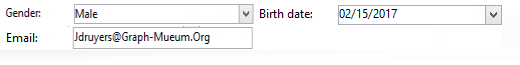``````<form name="recipient" namespace="cus">
<container colcount="2">
<input xpath="@gender"/>
<input xpath="@birthDate"/>
<input xpath="@email"/>
</container>
</form>
``````

`<container>` 元素與 colcount attribute可讓您將子控制項強制顯示在兩欄上。

colspan 控制項上的屬性會以值中輸入的欄數來擴充控制項：``````<form name="recipient" namespace="cus">
<container colcount="2">
<input xpath="@gender"/>
<input xpath="@birthDate"/>
<input xpath="@email" colspan="2"/>
</container>
</form>
````````````<form name="recipient" namespace="cus">
<container colcount="2" type="frame" label="General">
<input xpath="@gender"/>
<input xpath="@birthDate"/>
<input xpath="@email" colspan="2"/>
</container>
</form>
``````

A `<static>` 元素可用於格式化輸入表單：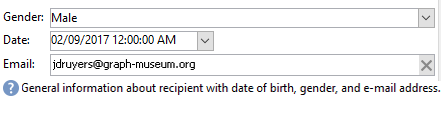``````<form name="recipient" namespace="cus">
<static type="separator" colspan="2" label="General"/>
<input xpath="@gender"/>
<input xpath="@birthDate"/>
<input xpath="@email" colspan="2"/>
<static type="help" label="General information about recipient with date of birth, gender, and email address." colspan="2"/>
</form>
``````

`<static>` 標籤為 分隔符號 type可讓您新增分隔列，其標籤包含在 標籤 屬性。

## 容器

xpath 上的屬性 `<container>` 可讓您簡化子控制項的參照。 然後，控制項的參照會相對於父項 `<container>` 元素。

``````<container colcount="2">
<input xpath="location/@zipCode"/>
<input xpath="location/@city"/>
</container>
``````

``````<container colcount="2" xpath="location">
<input xpath="@zipCode"/>
<input xpath="@city"/>
</container>
``````

### 容器型別

#### 索引標籤容器``````<container type="notebook">
<container colcount="2" label="General">
<input xpath="@gender"/>
<input xpath="@birthDate"/>
<input xpath="@email" colspan="2"/>
</container>
<container colcount="2" label="Location">
…
</container>
</container>
``````A style="down|up（依預設）" 特徵會強制標籤標籤垂直定位在控制項下方或上方。 此功能是選取性的。
`<container style="down" type="notebook"> … </container>`

#### 圖示清單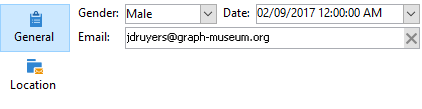``````<container type="iconbox">
<container colcount="2" label="General" img="xtk:properties.png">
<input xpath="@gender"/>
<input xpath="@birthDate"/>
<input xpath="@email" colspan="2"/>
</container>
<container colcount="2" label="Location" img="nms:msgfolder.png">
…
</container>
</container>
``````

#### 可見度容器

``````<container type="visibleGroup" visibleIf="@gender=1">
…
</container>
<container type="visibleGroup" visibleIf="@gender=2">
…
</container>
``````

• visibleIf="@email='peter.martinezATneeolane.net'"：測試字串型別資料的相等性。 比較值必須以引號括住。
• visibleIf="@gender >= 1和@gender！= 2英吋：數值的條件。
• visibleIf="@boolean1=true or @boolean2=false"：在布林值欄位上進行測試。

#### 正在啟用容器

``````<container type="enabledGroup" enabledIf="@gender=1">
…
</container>
<container type="enabledGroup" enabledIf="@gender=2">
…
</container>
``````

``````<element label="Company" name="company" target="cus:company" type="link"/>
``````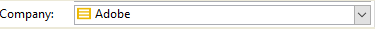``````<input xpath="company"/>
``````Edit link （放大鏡）圖示會啟動連結元素的編輯表單。 根據預設，使用的形式是在目標架構的索引鍵上推斷。 此 表單 屬性可讓您強制使用編輯表單的名稱（例如「cus：company2」）。

``````<input xpath="company">
<sysFilter>
<condition expr="[location/@city] =  'Newton"/>
</sysFilter>
</input>
``````

``````<input xpath="company">
<orderBy>
<node expr="[location/@zipCode]"/>
</orderBy>
</input>
``````

### 控制項屬性

• noAutoComplete：停用預先輸入（值為「true」）

• createmode：如果連結不存在，則會即時建立。 可能的值包括：

• ：停用建立。 如果連結不存在，則會顯示錯誤訊息
• 內嵌：在編輯欄位中建立與內容的連結
• 版本：在連結上顯示編輯表單。 驗證表單時，資料會儲存（預設模式）
• noZoom：連結上沒有編輯表單（值為「true」）

• 表單：多載目標元素的編輯表單

``````<element label="Events" name="rcpEvent" target="cus:event" type="link" unbound="true">
…
</element>
```````````` <input xpath="rcpEvent" type="linklist">
<input xpath="@label"/>
<input xpath="@date"/>
</input>
``````

Detail 按鈕會在以下情況自動新增： zoom="true" 屬性完成於 `<input>` 清單的標籤：可讓您啟動所選行的編輯表單。

`````` <input xpath="rcpEvent" type="linklist">
<input xpath="@label"/>
<input xpath="@date"/>
<sysFilter>
<condition expr="@type = 1"/>
</sysFilter>
<orderBy>
<node expr="@date" sortDesc="true"/>
</orderBy>
</input>
``````

### 關係表

``````<srcSchema name="subscription" namespace="cus">
<element name="service" type="link" target="cus:service" label="Subscription service"/>
</srcSchema>
``````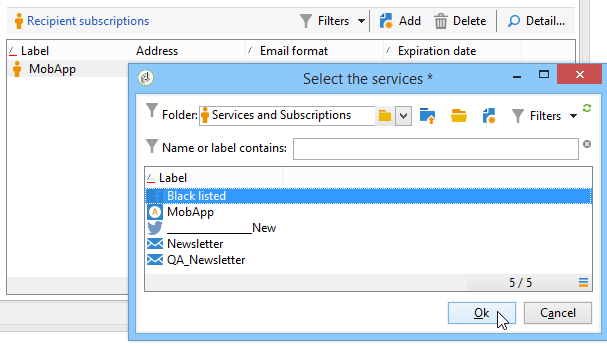``````<input type="linklist" xpath="subscription" xpathChoiceTarget="service" xpathEditTarget="service" zoom="true">
<input xpath="recipient"/>
<input xpath="service"/>
</input>
``````

Xpatchoicetarget 屬性可讓您從輸入的連結啟動選擇表單。 建立關係表記錄會自動更新目前收件者和所選服務的連結。

xpathEditTarget 屬性可讓您強制編輯所輸入連結上選取的行。

### 清單屬性

• noToolbar：隱藏工具列（值為「true」）
• toolbarCaption：多載工具列標籤
• toolbarAlign：修改工具列的垂直或水準幾何（可能的值： "vertical"|"horizontal"）
• img：顯示與清單關聯的影像
• 表單：多載目標元素的編輯表單
• 縮放：新增 Zoom 按鈕以編輯目標元素
• xpathEditTarget：在輸入的連結上設定編輯
• Xpatchoicetarget：此外，會在輸入的連結上啟動選取表單

## 記憶體清單控制項

### 欄清單``````<input xpath="rcpEvent" type="list">
<input xpath="@label"/>
<input xpath="@date"/>
</input>
````````````<input nolabel="true" toolbarCaption="List of events" type="list" xpath="rcpEvent" zoom="true">
<input xpath="@label"/>
<input xpath="@date"/>
</input>
``````

toolbarCaption attribute會強制水準對齊工具列，並輸入清單上方的標題。

#### 放大清單``````<input nolabel="true" toolbarCaption="List of events" type="list" xpath="rcpEvent" zoom="true" zoomOnAdd="true">
<input xpath="@label"/>
<input xpath="@date"/>

<form colcount="2" label="Event">
<input xpath="@label"/>
<input xpath="@date"/>
</form>
</input>
``````

### 清單屬性

• noToolbar：隱藏工具列（值為「true」）
• toolbarCaption：多載工具列標籤
• toolbarAlign：修改工具列的位置（可能的值： 「垂直」|「水準」）
• img：顯示與清單關聯的影像
• 表單：多載目標元素的編輯表單
• 縮放：新增 Zoom 按鈕以編輯目標元素
• Xpatchoicetarget：此外，會在輸入的連結上啟動選取表單

## 不可編輯的欄位

「性別」欄位範例：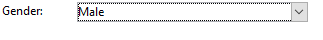``````<value value="@gender"/>
``````

## 選項按鈕

「性別」欄位範例：

``````<input type="RadioButton" xpath="@gender" checkedValue="0" label="Choice 1"/>
<input type="RadioButton" xpath="@gender" checkedValue="1" label="Choice 2"/>
<input type="RadioButton" xpath="@gender" checkedValue="2" label="Choice 3"/>
``````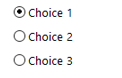## 核取方塊

``````<input xpath="@boolean1"/>
<input xpath="@field1" type="checkbox" checkedValue="Y"/>
``````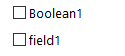## 分項清單

``````<input nolabel="true" type="treeEdit">
<container label="Text fields">
<input xpath="@text1"/>
<input xpath="@text2"/>
</container>
<container label="Boolean fields">
<input xpath="@boolean1"/>
<input xpath="@boolean2"/>
</container>
</input>
``````## 運算式欄位

``````<!-- Example: updating the boolean1 field from the value contained in the field with path /tmp/@flag -->
<input expr="Iif([/tmp/@flag]=='On', true, false)" type="expr" xpath="@boolean1"/>
<input expr="[/ignored/@action] == 'FCP'" type="expr" xpath="@launchFCP"/>
``````

## 表單的內容

### 更新內容

• `<set expr="'Test'" xpath="/tmp/@test" />`：將’Test’值放置在暫時位置/tmp/@test1
• `<set expr="'Test'" xpath="@lastName" />`：以「Test」值更新「lastName」屬性上的實體
• `<set expr="true" xpath="@boolean1" />`：將「boolean1」欄位的值設為「true」
• `<set expr="@lastName" xpath="/tmp/@test" />`：以「lastName」屬性的內容更新

``````<form name="recipient" namespace="cus">
<enter>
<set…
</enter>
…
<leave>
<set…
</leave>
</form>
``````

`<enter>``<leave>` 標籤可用於 `<container>` （「notebook」和「iconbox」型別）。

### 運算式語言

`<if expr="<expression>" />` 如果運算式已驗證，標籤會執行標籤下指定的指示：

``````<if expr="([/tmp/@test] == 'Test' or @lastName != 'Doe') and @boolean2 == true">
<set xpath="@boolean1" expr="true"/>
</if>
``````

`<check expr="<condition>" />` 標籤與 `<error>` 標籤會阻止表單驗證，如果不滿足條件，則會顯示錯誤訊息：

``````<leave>
<check expr="/tmp/@test != ''">
<error>You must populate the 'Test' field!</error>
</check>
</leave>
``````

## 精靈

``````<form type="wizard" name="example" namespace="cus" img="nms:rcpgroup32.png" label="Wizard example" entity-schema="nms:recipient">
<container title="Title of page 1" desc="Long description of page 1">
<input xpath="@lastName"/>
<input xpath="comment"/>
</container>
<container title="Title of page 2" desc="Long description of page 2">
…
</container>
…
</form>
``````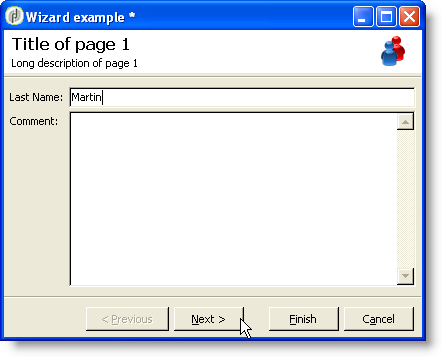Finish 按鈕儲存輸入的資料並關閉表單。

### SOAP方法

SOAP方法執行可從填入的啟動 `<leave>` 標籤的後面。

`<soapcall>` 標籤包含方法的呼叫，並具有下列輸入引數：

``````<soapCall name="<name>" service="<schema>">
<param  type="<type>" exprIn="<xpath>"/>
…
</soapCall>
``````

• 字串：字元字串
• 布林值：布林值
• 位元組：8位元整數
• ：16位元整數
• ：32位元整數
• ：16位元整數
• 兩次：雙精確度浮點數
• DOMElement：元素型別節點

exprIn attribute包含要以引數形式傳遞的資料位置。

``````<leave>
<soapCall name="RegisterGroup" service="nms:recipient">
<param  type="DOMElement"    exprIn="/tmp/entityList"/>
<param  type="DOMElement"    exprIn="/tmp/choiceList"/>
<param  type="boolean"       exprIn="true"/>
</soapCall>
</leave>
``````